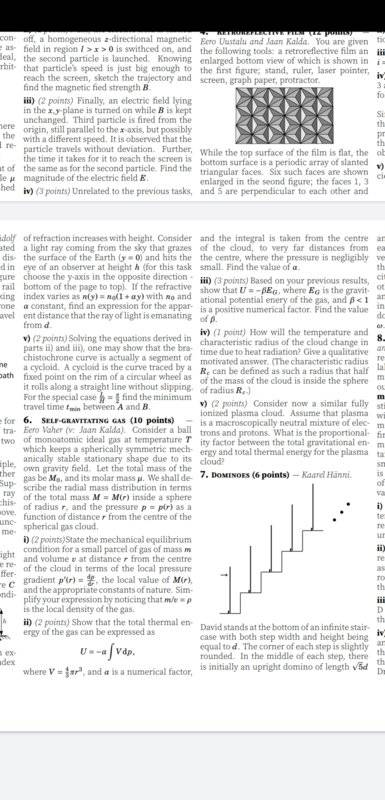# Spherical gas distributed symmetricaly over space

Homework Statement:
Since the problem is long, and my doubt is very specific, I'll show it in a picture, it is the problem 6. But summing up, the question is in the item ii) where it is asked to calculate the total thermal energy of the gas.
Relevant Equations:
U=nCvT ; PV=nRT;.

We have that energy in a infinitesimal Spherical layer with number of mols dn is:
dU=Cv.T.dn (1)
But by the ideal gas law:
PV=nRT (2)
Differentiation gives:
PdV+VdP=RTdn (3)
(3) in (1) and using CV=3R/2 (monoatomic)
gives:
dU=3/2.(PdV+VdP) (4)
Integration of (4) over the whole gas will not give the result expected. Where is my mistake?

Homework Helper
Gold Member
2020 Award
For (2) ##PV=nRT ##, the ## n ## in that formula is the total moles in a volume ## V ## at pressure ## P ## and temperature ## T ##. Changing the number of moles by ## dn ## at temperature ## T ##, (##dn ## uniformly distributed throughout the volume ##V ##), will result in the formula you obtained when you differentiated both sides. The integration of both sides of the equation, where you are completely changing the scenario to which the equation applies, is simply invalid.

Last edited:
You say that equation 3 is valid, but it is not valid to integrate it over the whole volume ? This change of the scenario would be caused by what? Is it because of the gradient pressure, like if you were assuming all gas to be at the same thermodynamic equilibrium?

•